Mat 331

Exercise 3: Some Elementary Maple

1. Evaluate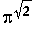to 30 decimal places.

> evalf(Pi^(sqrt(2)),30);2. Consider the function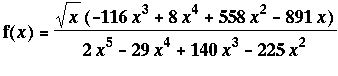> f:= x-> (sqrt(x)*(-116*x^3+8*x^4+558*x^2-891*x))/
(2*x^5-29*x^4+140*x^3-225*x^2);a. Write this in a simpler form (that is, factor and reduce it).

> factor(f(x));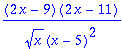b. Draw the graph of the function for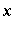between 0 and 10. Adjust the vertical range so some detail can be seen.

> plot(f(x),x=0..10);That looks terrible (too much range is wasted on the asymptote at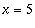), so lets try looking at a restricted range, as suggested. After a bit of playing around, it seems that the following works well, indicating both the asymptotes at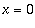and, but also some of the detail when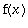is between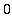andor so.

> plot(f(x),x=0..10,y=-10..10);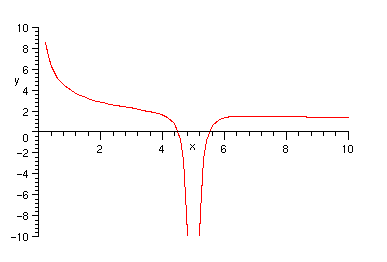c. Compute the area of the part of the curve that lies above the-axis and between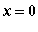and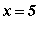(i.e., integrate the function over the appropriate range ofvalues). Give your answer both in an exact form and as a decimal approximation to about 10 places.

First, we need to notice where the function crosses the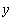-axis. This is easily seen to be wherefrom the answer to part a , but we could also let maple do all the work:

> solve(f(x)=0,x);Now that we have that, the rest is straightforward.

> int(f(x),x=0..9/2);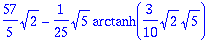> evalf(%);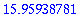d. What is the value of the integral if you remove the factor of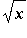from the numerator?

This is most simply expressed as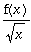, but of course there are many other ways to do it.

> int(f(x)/sqrt(x),x=0..9/2);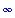The answer is infinite, because the new function looks like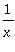near, which is does not bound a finite area (unlike).

e. Use the derivative ofto determine for what realthe function has a local maximum. An approximation to about 8 decimal places is sufficient.

We can do this all in one maple statement, supressing all the intermediate messiness. Note that we don't care what the derivative is, only where it is zero. Also, we know from the graph in part b that the answer is about 6, and that it is clearly a local maximum, so we needn't mess with second derivatives, although we could if we really wanted to.

> evalf( solve ( diff(f(x),x)=0, x));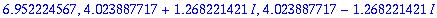There are three critical points, but only one of them is a real number; the others are complex. So the maximum occurs at

> [6.952224567, f(6.952224567)];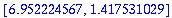3. Use the commands seq and ithprime generate a list of the first 20 primes. Compute the sum of the first 20 primes, and give its integer factors.

> seq(ithprime(i),i=1..20);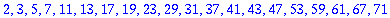> sumprimes:= sum(ithprime(i),i=1..20);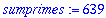> ifactor(sumprimes);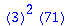4. Find the solutions to the system of equations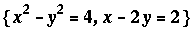.

> solve({x^2-y^2=4, x-2*y=2},{x,y});We should, of course, expect two solutions, because this is the intersection of a hyperbola and a line.

> plots[implicitplot]({x^2-y^2=4, x-2*y=2},x=-4..4,y=-4..4);>

5. Draw a graph showing both cos(x) and its fifth taylor polynomial (that is,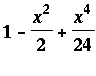) for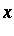between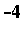and 4. How many terms do you seem to need to get ``good agreement'' in this range (you will need to use something like convert(taylor(cos(x),x,5), polynom) to make this work. You should think about an appropriate way to demonstrate that the approximation you've taken is ``good''.

Note that the command taylor() gives us an object which is a series, rather than a polynomial, that is, it has an order of trunctation with it. Thus the result of taylor() cannot be plotted directly:

> taylor(cos(x),x,5);> plot(%);

Plotting error, empty plot

So, to get around that, we can use convert() to discard theterm and give us a polynomial.

Here are the two functions together:

> plot({cos(x),convert(taylor(cos(x),x,5), polynom)},x=-4..4);Now, we could just keep making that picture, increasing the number of terms by two (every other term in the taylor series of cosine is 0) until it looks good. But, we might as well be a bit fancier and do it all at once. Since I will need it later, I am also going to define a function which gives me the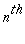series.

> nthseries := n -> convert( taylor(cos(x),x,n), polynom);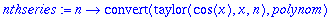Now I will plot the cosine and several of the approximations on the same graph, and see which looks good. I'll use seq() to generate them. (Note that since I only want odd numbers, I will index them by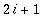so the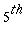polynomial will correspond to i=2. That is, we are giving the number of non-constant terms rather than the order).

We can see a bit better what is going on if we look at a slightly different domain and range.

> plot([cos(x), seq(nthseries(2*i+1),i=1..7)],x=0..6,y=-1..1);Note that all of the series fit well near x=0, but the last two (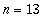and) have graphs which are visually indistinguishable from the cosine while. The graph below demonstrates thatgives a "good" fit over the desired range, since it seems we are plotting only one function.

> plot([cos(x), nthseries(13)],x=-4..4);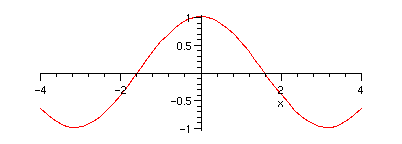However, plotting the difference of the two is a better indication of the goodness of fit:

> plot(nthseries(13)-cos(x),x=-4..4);Truncating at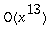gives us agreement over the whole range to within 0.003, which is pretty good.

There are, of course, many other measures of "good".

>